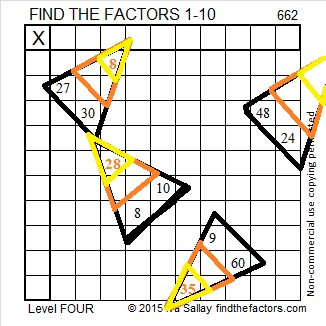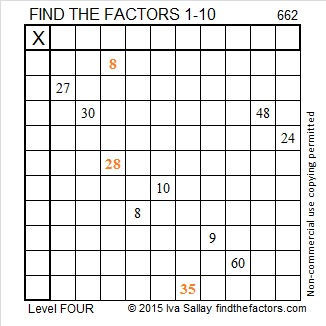# 662 More Candy Corn

662 is the sum of the twelve prime numbers from 31 to 79.Print the puzzles or type the solution on this excel file: 10 Factors 2015-10-26

Here’s the same candy corn puzzle but less colorful.—————————————————————————————————

• 662 is a composite number.
• Prime factorization: 662 = 2 x 331
• The exponents in the prime factorization are 1 and 1. Adding one to each and multiplying we get (1 + 1)(1 + 1) = 2 x 2 = 4. Therefore 662 has exactly 4 factors.
• Factors of 662: 1, 2, 331, 662
• Factor pairs: 662 = 1 x 662 or 2 x 331
• 662 has no square factors that allow its square root to be simplified. √662 ≈ 25.72936.—————————————————————————————————Ricardo tweeted the solution to the puzzle so I’m including it here as well.